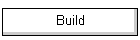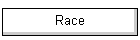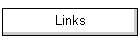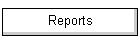The collection of spreadsheets here takes an arbitrary shape and turns it into a sail section.  Might be useful if you are thinking of making your own sails, or investigating the aerodynamics of sail sections.  The first part of the page discusses the "parabola" spreadsheet in some detail.  The later part of the page introduces the circular arc and bi-circular arc spreadsheets in less detail, since they are almost identical to the parabola version.  This graphic shows the ways in which these three sections are similar, and different.  Draft is 10% for all three sections.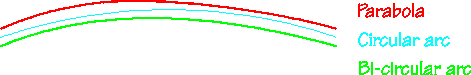The parabola spreadsheet (88 kb), now in version 2, takes a segment of a parabola and translates, rotates, and scales it to become a sail section. You can play with the parabola to generate a sail shape with different max draft, and different position of max draft.  It then calculates various parameters of the section shape, including girth, entry and exit angles, radius of curvature at entry, exit, and point of max draft, angle of zero lift, sail force direction, and estimated maximum coefficient of lift.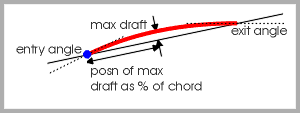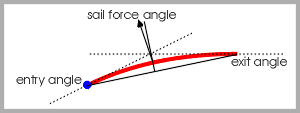The spreadsheet calculates 103 points along the chosen parabolic arc, and plots the middle 101 points to give an idea of the shape of the arc.  The first and last points are used to calculate the tangent at entry and exit, and hence the entry and exit angles.  For each point, the estimated radius of curvature "r" is calculated, as is the angle this radius makes with the arc focus, "Theta".  The "x" and "y" coordinates of the arc are then calculated, and plotted on a small graph to the right of the spreadsheet page. The raw parabolic arc must then be translated, rotated, and scaled to become a sail section.  First, the arc is moved so that one or other of its ends starts at the point (0,0).  The x and y values are translated into new x' and y' values by subtracting the required translation x and y values shown.  Then, the arc is rotated around the (0,0) point so that it lies horizontally.  The rotation is given by the formula x'' = x' sin(Phi) + y'cos(Phi), y'' = y'cos(Phi) - x'sin(Phi), where Phi is the required angle of rotation.  Finally, the arc is scaled so that its chord matches the value specified in the "desired chord" spreadsheet cell.  The value of the scaling multiplier "s" is shown, where x''' = s.x'' and y''' = s.y''. The spreadsheet then scans the 101 section points to find the point at which the depth of the sail is a maximum (maximum y), the position along the chord at which this maximum occurs (corresponding x), and the radius of curvature (corresponding r).  These values are used to calculate the various section characteristics.Note that the "desired chord" is not quite the measured foot of the sail.  The chord is the foot of the sail as set on the boom, complete with the draft set using the outhaul.  When the sail is measured, of course, the foot is stretched and the draft taken out to yield the true sail foot or "girth".  The amount that the girth differs from the chord is the "sag", the amount you adjusted the outhaul to put the draft into the sail.  If you wanted to plot the points for the foot of an IOM No.1 mainsail with 10% draft, say, then you need to set a chord of around 345. The entry and exit angles are calculated in three different ways.  The first way estimates these angles by taking the tangent at the points numbered -1 and 1 for the entry, and the points numbered 99 and 101 for the exit.  The two other ways use formulas.  One formula takes advantage of the fact that light rays are all reflected to the focus of a parabola, and so the tangent at any point is half the value of Theta, the angle the radius makes with the focus.  The other formula uses the fact that the tangent vector for a parabola is given by x = 1/sqrt(1+t^2), y=t/sqrt(1+t^2).  In the example parabolic arc shown in the screen shot, max draft is 10%, positioned at 45% of chord.  This yields an entry angle of about 26 or 27 degrees. The angle of zero lift is useful for theoretical aerofoil analysis, and is estimated in a number of ways.  The first, and probably the most reliable way, uses a method of Pankhurst (Abbott & von Doenhoff, p.72).  The second method uses the simple formula -2m, where m is the % of max draft.  The third and fourth methods are described in Abbott & von Doenhoff, p.71, and yield two versions of the Theodorsen analysis.  The angle is calculated in radians, and the spreadsheet converts radians to degrees for presentation.  The Pankhurst and Theodorsen methods require the use of coefficients at various stations along the chord. The estimated maximum coefficient of lift of the section is given by considering that the sail gives its max lift when its entry angle equals the angle of incidence of the local wind.  Theoretically, the coefficient of lift is given by the formula "2.pi(alpha + 2m)", but in practice this seems to be more like "pi(alpha + 2m)". "Alpha" is the angle of attack of the sail, measured relative to the chord. Note that this estimate is only near the money for flatter sails, say up to 6% draft. Fuller sails stall well before they get to their theoretical maximum angle of attack, for reasons I don't understand, but which may have something to do with the induced downwash increasing with coefficient of lift. Finally, the direction in which the sail force acts is given by the bisection of the entry and exit angles.  As long as max draft is forward of 50% of chord, entry angle is larger than exit, and the sail force is directed forwards by a modest angle.  The more this force is directed forward, the more drive the sail gives.  This is one of reasons why a draft-forward sail gives more drive when beating than a draft-aft sail. There are two more spreadsheets which could be useful.  One uses a circular arc section (about 86 kb), and the other uses a bi-circular arc section (85 kb).The circular arc section was studied in early writing on theoretical aerodynamics.  More recently it is more or less impossible to find anyone testing such a section in a wind tunnel.  It was a useful section because it has well-known characteristics.The bi-circular arc spreadsheet makes up a sail section from two separate circular arcs, a first or forward arc, and a second or aft arc.  This allows the section to have maximum draft forward of 50% of chord (where it is fixed, of course, by a simple circular arc section).  A little careful juggling is needed to get the section you want, but the basic idea is to have a smaller radius arc at the forward part of the section, and to have more of the spreadsheet's "theta" used forward.  Then, a larger radius arc is set as the aft part of the section, with less of the "theta" used aft.  The spreadsheet's  "theta" is split at the half-pi position, 1.571 radians.  The forward theta might start at 2.1 radians, as in the example screen shot, and so run down to 1.571, while the aft theta runs from 1.571, in this example, down to 1.305.  The result is a section with 10% draft, placed at 40.1% of chord.  It is very similar to the parabolic section shown earlier. 2018-06-22